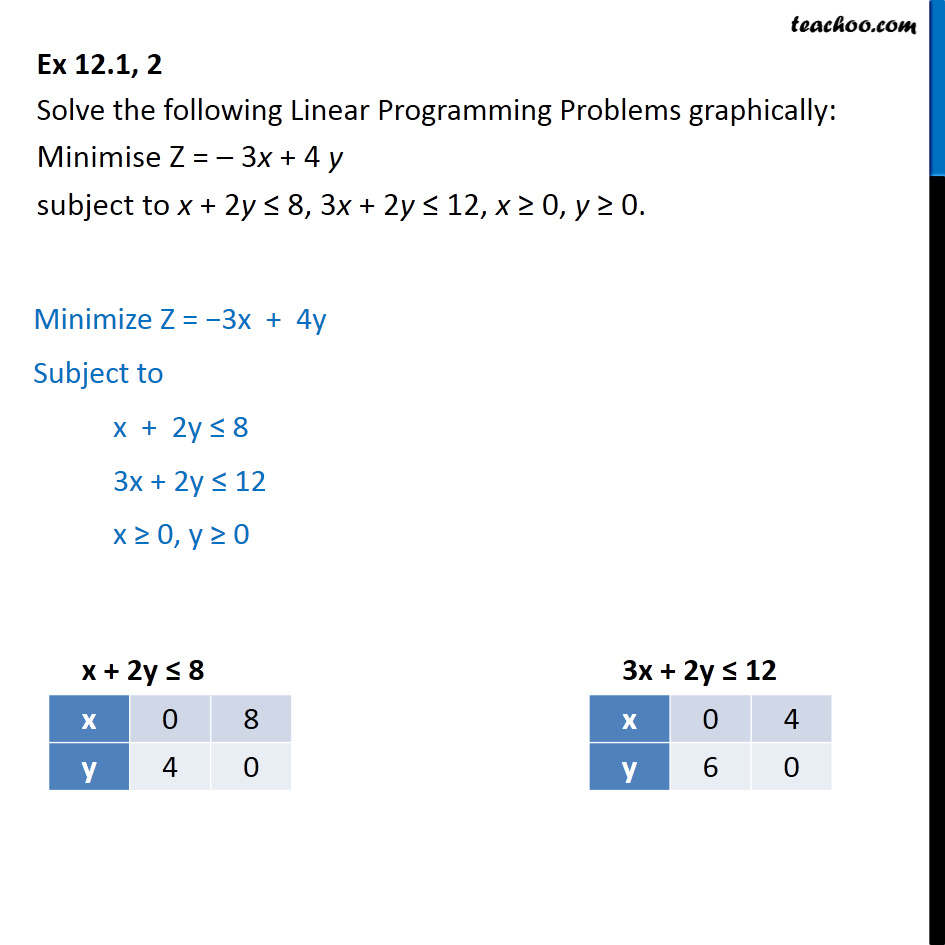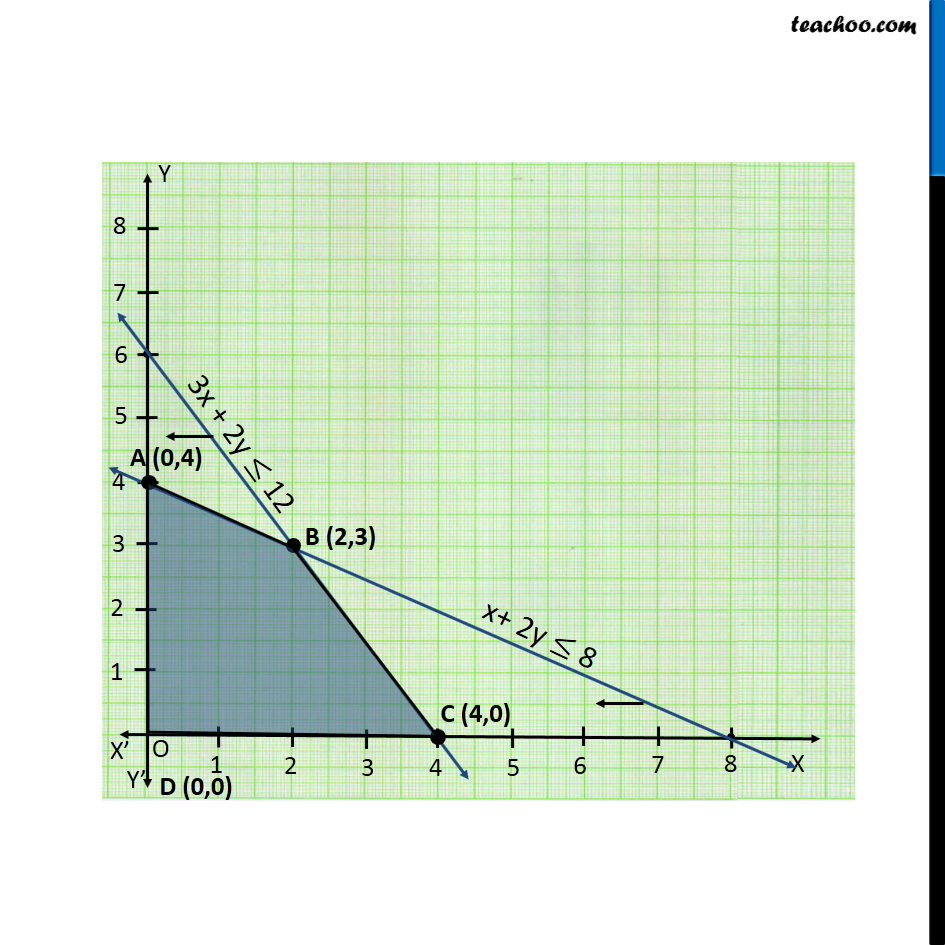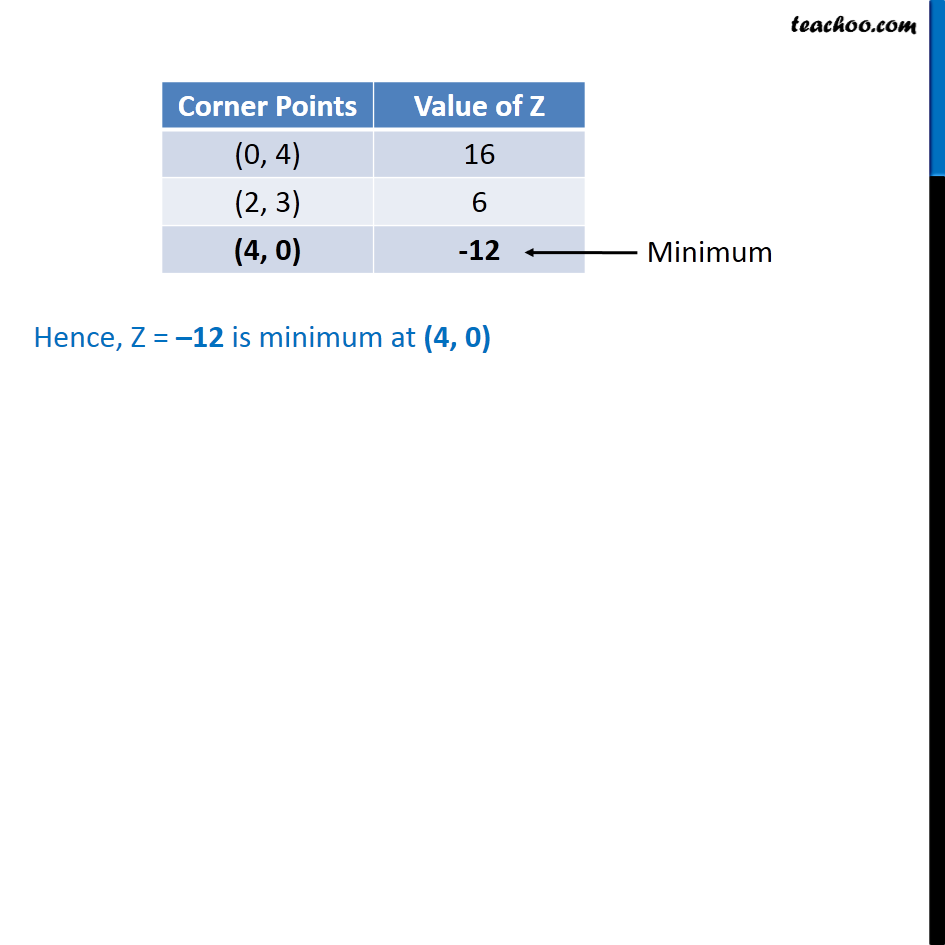Linear equations given - Bounded

Chapter 12 Class 12 Linear Programming
Concept wiseIntroducing your new favourite teacher - Teachoo Black, at only ₹83 per month

### Transcript

Ex 12.1, 2 Solve the following Linear Programming Problems graphically: Minimise Z = – 3x + 4 y subject to x + 2y ≤ 8, 3x + 2y ≤ 12, x ≥ 0, y ≥ 0. Minimize Z = −3x + 4y Subject to x + 2y ≤ 8 3x + 2y ≤ 12 x ≥ 0, y ≥ 0 Hence, Z = –12 is minimum at (4, 0)